Next: Quadratic Stark Effect Up: Time-Independent Perturbation Theory Previous: Two-State System

# Non-Degenerate Perturbation Theory

Let us now generalize our perturbation analysis to deal with systems possessing more than two energy eigenstates. Consider a system in which the energy eigenstates of the unperturbed Hamiltonian,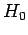, are denoted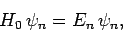(894)

where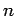runs from 1 to. The eigenstates are assumed to be orthonormal, so that(895)

and to form a complete set. Let us now try to solve the energy eigenvalue problem for the perturbed Hamiltonian: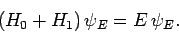(896)

If follows that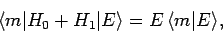(897)

wherecan take any value from 1 to. Now, we can expressas a linear superposition of the unperturbed energy eigenstates: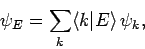(898)

whereruns from 1 to. We can combine the above equations to give(899)

where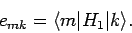(900)

Let us now develop our perturbation expansion. We assume that(901)

for all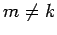, where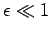is our expansion parameter. We also assume that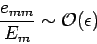(902)

for all. Let us search for a modified version of theth unperturbed energy eigenstate for which(903)

and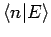(904)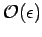(905)

for. Suppose that we write out Eq. (899) for, neglecting terms which areaccording to our expansion scheme. We find that(906)

giving(907)

Substituting the above expression into Eq. (899), evaluated for, and neglecting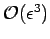terms, we obtain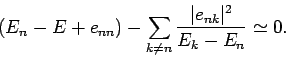(908)

Thus, the modifiedth energy eigenstate possesses an eigenvalue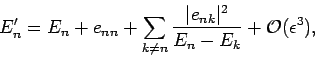(909)

and a wavefunction(910)

Incidentally, it is easily demonstrated that the modified eigenstates remain orthonormal to.Next: Quadratic Stark Effect Up: Time-Independent Perturbation Theory Previous: Two-State System
Richard Fitzpatrick 2010-07-20